## Physics

Question 1.
Figure shows a capacitor made of two circular plates each of radius 12 cm, and separated by 5.0 mm. The capacitor is being charged by an external source (not shown in the figure). The charging current is constant and equal to 0.15 A.(a) Calculate the capacitance and the rate of change of potential difference between the plates.
(b) Obtain the displacement current across the plates.
(c) Is Kirchhoff’s first rule (junction rule) valid at each plate of the capacitor? Explain.
Solution:

(a) Capacitance of the parallel plate capacitor(b) The magnitude of displacement current is equal to conduction current. ∴ Id = I = 0.15 A
(c) Yes, Kirchhoff’s first law is very much applicable to each plate of capacitor as ld = I. So current is continuous and constant across each plate.Question 2.
A parallel plate capacitor as shown in figure made of circular plates each of radius R = 6.0 cm has a capacitance C = 100 pF. The capacitor is connected to a 230 V ac supply with a (angular) frequency of 300 rad s-1.
(a) What is the rms value of the conduction current?
(b) Is the conduction current equal to the displacement current?
(c) Determine the amplitude of 6 at a point 3.0 cm from the axis between the plates.Solution:
(a) Capacity of capacitor C = 100 pF(b) Yes, the conduction current in wires is always equal to displacement current within plates.
(c)To find magnetic field B of a point 3 cm from the axis within plates, let us assume a loop of radius 3 cm with center on axis. Now modified Ampere’s Law.Question 3.
What physical quantity is same for X-rays of wavelength 10-10 m, red light of wavelength 6800 A and radio waves of wavelength 500 m?
Solution:
Though they all have different wavelengths and frequencies, but they have same speed i.e., speed of light 3 × 108 m s-1 in vacuum.

Question 4.
A plane electromagnetic wave travels in vacuum along z-direction. What can you say about the directions of its electric and magnetic field vectors? If the frequency of the wave is 30 MHz, what is its wavelength?
Solution:
In electromagnetic wave, the electric field vector E and magnetic field vector B show their variations perpendicular to the direction of propagation of wave as well as perpendicular to each other. As the electromagnetic wave is travelling along z-direction, hence E and B show their variation in x-y plane.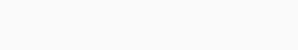Question 5.
A radio can tune in to any station in the 7.5 MHz to 12 MHz band. What is the corresponding wavelength band?
Solution:
Maximum wavelength in the band is for lowest frequencyMinimum wavelength in the band is for highest frequencyQuestion 6.
A charged particle oscillates about its mean equilibrium position with a frequency of 109 Hz. What is the frequency of electromagnetic wave produced by the oscillator?
Solution:
The frequency of electromagnetic wave produced will be same as the frequency of oscillation of charged particles i.e., 109 Hz.

Question 7.
The amplitude of the magnetic field part of a harmonic electromagnetic wave in vacuum is B0 = 510 nT. What is the amplitude of the electric field part of the wave?
Solution:

Question 8.
Suppose that the electric field amplitude of an electromagnetic wave is E0 = 120 N C-1 and that its frequency is u = 50.0 MHz. (a) Determine B0, to, k, and X.
(b) Find expressions for E and B.
Solution:
(a) By relation, E0B0=c(b) Let the wave is propagating along A’-direction, electric field E is along y – direction and magnetic field B along z-direction.Question 9.
The terminology of different parts of the electromagnetic spectrum is given in the NCERT text. Use the formula E = hυ and obtain the photon energy in units of eV for different parts of the electromagnetic spectrum. In what way are the different scales of photon energies that you obtain related to the sources of electromagnetic radiation?
Solution:
The photon of given energy are released during transition between energy levels in the atom and the emitted photon energy is equal to difference of energies of energy levels, among which the transition has taken place. For example photon energy for
λ = 1 m can be calculatedSo, for emission of radio waves the energy difference between energy levels should be 12.425 × 10-7 eV. Similarly, photon energy for other wavelengths can also be calculatedSo, for emission of y -rays the energy difference among energy levels should be of the order of MeV, where for visible radiation it should be of the order of eV.

Question 10.
In a plane electromagnetic wave, the electric field oscillates sinusoidally at a frequency of 2.0 × 1010 Hz and amplitude 48 Vm-1.
(a) What is the wavelength of the wave?
(b) What is the amplitude of the oscillating magnetic field?
(c) Show that the average energy density of the E field equals the average energy density of the B field, [c = 3 × 108 m s-1.]
Solution:
(a) Wavelength of electromagnetic wave is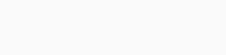(b) Amplitude of magnetic field, E0B0=c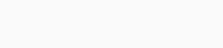(c) Energy density as electric field,Question 11.
Suppose that the electric field part of an electromagnetic wave in vacuum is £ = [(3.1 N/C)] cos[(1.8 rad/m) y + (5.4 x 108 rad/s)f)] i.
(a) What is the direction of propagation?
(b) What is the wavelength λ?
(c) What is the frequency υ?
(d) What is the amplitude of the magnetic field part of the wave?
(e) Write an expression for the magnetic field part of the wave.
Solution:
(a) Electromagnetic wave is propagating in -y direction.
(b) Propagation constant, k = 2πλ = 1.8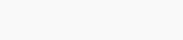(c) angular frequency, ω = 2πυ = 5.4 × 108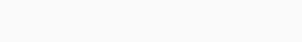(d) Amplitude of magnetic field pat, E0B0=c(e) Expression for magnetic field pat of wave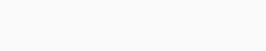Question 12.
About 5% of the power of a 100 W light bulb is converted to visible radiation. What is the average intensity of visible radiation
(a) at a distance of 1 m from the bulb?
(b) at a distance of 10 m?
Assume that the radiation is emitted is o tropically and neglect reflection.
Solution:
The bulb as a point source, radiate light in all direction uniformly and it is given that only 5% of power is converted to visible radiations.
(a) Let us assume a sphere of radius 1 m. Surface area A = 4πr2 = 4π (l)2 = 4 π m2 Intensity on the sphere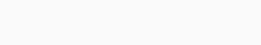(b) Let us assume a sphere of 10 m radius. Surface area A = 4π2 = 4K (10)2 = 400 π m2 Intensity on the sphere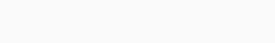Question 13.
Use the formula λmT = 0.29 cm K to obtain the characteristic temperature ranges for different parts of the electromagnetic spectrum. What do the numbers that you obtain tell you?
Solution:
A black body at very high temperature produce a continuous spectrum. Using Wien’s displacement law we can calculate the wavelength corresponding to maximum intensity of radiation emitted Required absolute temperature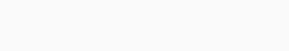Using above formula, the temperature of black body required for various wavelength is calculated.To produce electromagnetic radiations of different wavelength, we need temperature ranges. To produce visible ’ radiation of X = 5 × 10-7 m, we need to have source at temperature of 6000 K. A source at lower temperature will produce this wavelength but not with maximum intensity.

Question 14.
Given below are some famous numbers associated with electromagnetic radiations in different contexts in Physics. State the part of the electromagnetic spectrum to which each belongs.
(a) 21 cm [wavelength emitted by atomic ‘hydrogen in interstellar space].
(b) 1057 MHz [frequency of radiation arising from two close energy levels in hydrogen; known as Lamb shift].
(c) 2.7 K [temperature associated with the isotropic radiation filling all space-thought to be a relic of the ‘big-bang’ origin of the universe].
(d) 5890 A – 5896 A [double lines of sodium]
(e) 14.4 keV [energy of a particular transition in 57Fe nucleus associated with a famous high resolution spectroscopic method (Mossbauer spectroscopy)].
Solution:
(a) 21 cm wavelength corresponds to radio waves, at nearly 1445 MHz.
(b) Electromagnetic wave of 1057 MHz also corresponds to radio waves.
(c) Using Wien’s Law the wavelength of the radiation mostly emitted at given temperature of heavenly bodies can be calculatedThis wavelength corresponds to microwave region of electromagnetic waves.
(d) This wavelength corresponds to visible region (yellow) of electromagnetic waves.
(e) The frequency of radiation can be calculatedThis frequency corresponds to X-ray region of electromagnetic waves.

Question 15.